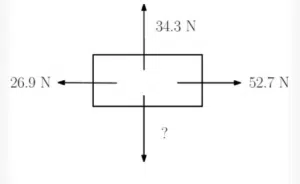# Newton’s Second Law of Motion

Newton’s Second Law of Motion states that when a non-zero resultant force is applied to an object, it causes the object to accelerate. In particular, the resultant force is proportional to the acceleration. More precisely, the resultant force is the product of the object’s mass and its acceleration.

### Newton’s Second Law of Motion:

The resultant force acting on a body is equal to its mass multiplied by its acceleration. Mathematically speaking,

Newton’s Second Law of Motion:
${\bf F}=m{\bf a}.$

Consider two objects with different masses. Specifically, object 1 has a much larger mass than object 2. From Newton’s Second Law we can see that the force required to give the objects the same acceleration will be much larger for object 1.

This can be seen in an episode of Brian Cox’s Human Universe. This episode features the world’s largest vacuum inside which a feather and a ball are dropped simultaneously. In the absence of air resistance, both objects have exactly the same acceleration. These objects are only being worked on by gravitational force, that is, mass multiplied by acceleration for each object. Since the mass is much higher for the ball, the force required to accelerate it is much larger too.

Newton’s Second Law is closely related to Newton’s First Law if you consider a resultant force of 0. When an object is either stationary or moving with constant velocity its acceleration is zero. It follows that the resultant force is zero (when zero acceleration is multiplied by non-zero mass). In other words, Newton’s Second Law is a more general statement about forces than Newton’s First Law.

Newton’s Second Law of Motion is the most used of Newton’s Laws and is often referred to as ‘The Law of Motion’.

## Newton's Second Law Examples

1. An object with mass 10kg accelerates at a rate of 2 m/s/s. What is the force acting on the object?
2. If a body of mass 80kg is acted on by a resultant force of 5N, what is the object’s acceleration?
3. What is the force required to give an object of mass 100g an acceleration of 5m/s/s?

The following object has only horizontal acceleration. Assuming that acceleration due to gravity is 9.8 m/s/s, find the object’s mass. Find the horizontal acceleration.A car of mass 1250kg accelerates at a rate of 3.7 m/s/s. If the thrust of the car is 12kN, calculate its drag.

An object has horizontal as well as vertical acceleration. This acceleration is caused by three forces. These are given by $3{\bf i}+4{\bf j}$, $-8{\bf i}+2{\bf j}$ and $5{\bf i}-3{\bf j}$. If the object has a mass of 10kg, find the horizontal and vertical acceleration.